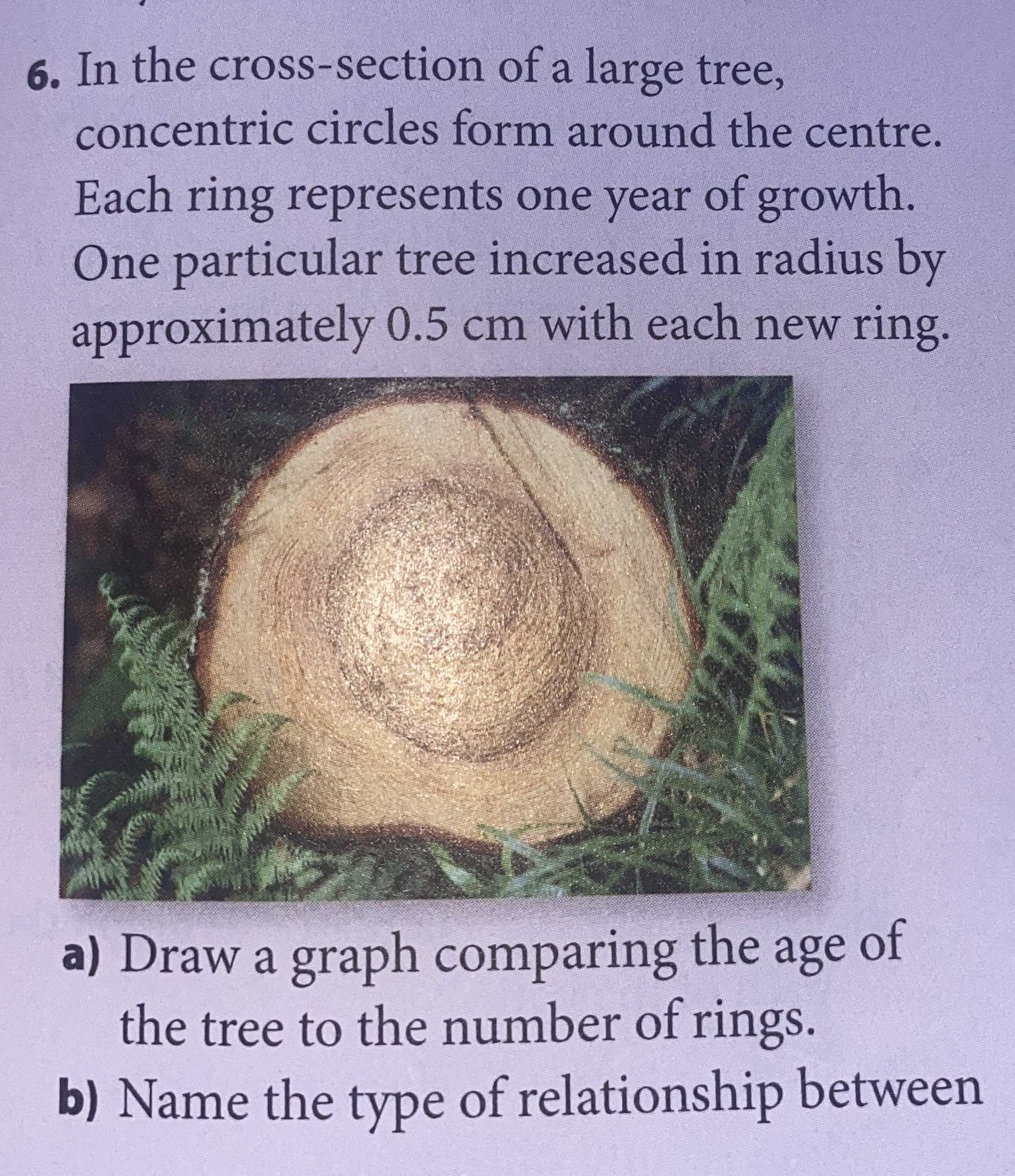### ¿Todavía tienes preguntas de matemáticas?

Pregunte a nuestros tutores expertos
Algebra
Pregunta6. In the cross-section of a large tree, concentric circles form around the centre. Each ring represents one year of growth. One particular tree increased in radius by approximately $$0.5 cm$$ with each new ring.

a) Draw a graph comparing the age of the tree to the number of rings.

b) Name the type of relationship between

(a)$$R= t$$
(b)According to 2 equations $$R= T$$ and $$T= t$$,they have linear relations.